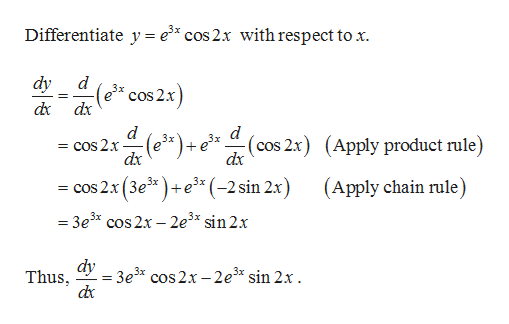# Verify that the indicated function is an explicit solution of the given differential equation. Assume an appropriate interval I. d^2y/dx^2 - 6dy/dx +13y =0 ; y=e^(3x)cos2x

Question
91 views

Verify that the indicated function is an explicit solution of the given differential equation. Assume an appropriate interval I.

d^2y/dx^2 - 6dy/dx +13y =0 ; y=e^(3x)cos2x

check_circle

Step 1

The function is given as:

Step 2

The differential equation is given as:

Step 3

To verify: The given function is the explicit solution o...help_outlineImage TranscriptioncloseDifferentiate y = e* cos2x with respect to x. dy d 3x cos 2x dx d d = cOs 2x dx d 3x +e(cos 2x(Apply product rule) dx (Apply chain rule) cos 2x (3e+e** (-2 sin 2x) = 3e3* cos2x -2e3* sin 2x Thus ay3e3* cos 2x-2e** sin 2x. fullscreen

### Want to see the full answer?

See Solution

#### Want to see this answer and more?

Solutions are written by subject experts who are available 24/7. Questions are typically answered within 1 hour.*

See Solution
*Response times may vary by subject and question.
Tagged in

### Math